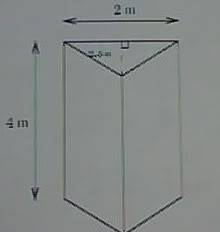# Density, Volume and Slicing Problem

Eonfluxx

## Homework Statement

A compressible liquid has density which varies with height. AT the level of h meters above the bottom, the density is 40(5 - h) kg/m3
a) The liquid is put in the containers below. The cross sections of the container are isosceles triangles. It has straight sides and looks like a triangular prism. How many kg will it hold when placed as shown on the left, resting on one triangular side?​^There is a picture of the object.

## Homework Equations

Mass = Volume times Density

## The Attempt at a Solution

My theory is that since M = V*D, and you're given the density, should I slice and solve for volume, then evaluate the integral of volume and the given integral for density then multiply the results to get the mass?
$$\int^{4}_{0}2.5 dh$$ and $$40\int^{4}_{0}5-h dh$$

I got to the first integral by taking the volume of the first slice (triangular prism):
$$\sum\frac{1}{2}b*l*\Delta h$$ $$\rightarrow$$ $$\sum\frac{1}{2}2*2.5\Delta h$$ $$\rightarrow$$ lim as $$\Delta h$$ $$\rightharpoonup$$$$\int^{4}_{0}2.5 dh$$

To find the mass, according to the equation M=VD, should I solve both integrals , one being V and one being D, then multiply?